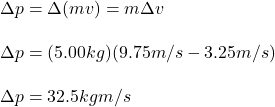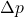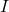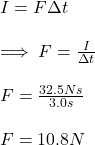## – A 5.00-kg body accelerates from 3.25 m/s to 9.75 m/s in 3.0 seconds when a force acts upon it. Find (a) the change in momentum

Question

A 5.00-kg body accelerates from 3.25 m/s to 9.75 m/s in 3.0 seconds when a force acts upon it. Find (a) the change in momentum of the body, (b) the impulse produced by the force, and (c) the magnitude of the force.​

in progress 0
2 months 2021-07-26T00:17:44+00:00 2 Answers 3 views 0

(a) the change in momentum of the body is 32.5 kg.m/s

(b) the impulse produced by the force is 32.5 N.s

(c) the magnitude of the force is 10.83 N

Explanation:

Given;

mass of the body, m =  5.00-kg

initial velocity of the body, u = 3.25 m/s

final velocity of the body, v = 9.75 m/s

time taken, t = 3.0 seconds

Part (a) the change in momentum of the body

ΔP = mv – mu

= (5 x 9.75) – (5 x 3.25)

= 48.75 – 16.25

= 32.5 kg.m/s

Part (b) the impulse produced by the force

I = f x t

where;

I is impulse

f is the applied force

t is time

f x t = mΔv

I = mΔv

I = m (v -u)

I = 5 (9.75 – 3.25)

I = 32.5 N.s

Part (c) the magnitude of the force;

I = f x t​

f = I / t

where;

f is magnitude of the force.

I is impulse

t is time

f = 32.5 / 3

f = 10.83 N

(a) 32.5 kgm/s

(b) 32.5 Ns

(c) 10.8 N

Explanation:

The change in momentum can be calculated from the definition of linear momentum:Then, the change in momentum of the body is of 32.5 kgm/s (a).

Now, from the impulse-momentum theorem, we know that the change in momentum of a bodyis equal to the impulseexerted to it. So, the impulse produced by the force equals 32.5 kgm/s (or 32.5 Ns) (b).

Finally, since we know the value of the impulse and the interval of time, we can easily solve for the magnitude of the force:It means that the magnitude of the force is of 10.8N (c).